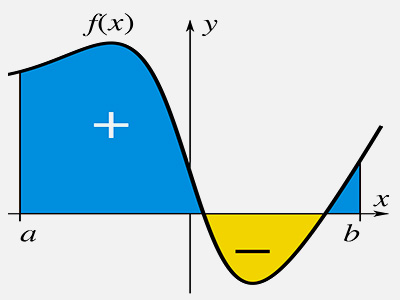ΓραμματείαSecretariat: 2410 684574 | ΦοιτητικάStudents: 2410 684387 g-ds@uth.gr
Select Page

# Numerical Analysis

Υ301

3

#### Hours/Week - ECTS

5 – 5### Learning Outcomes

Upon successful completion of the course, the student will:

• Have understood ways of solving linear systems by direct and iterative methods and will be able to judge which is the appropriate method to use for the given problem.
• Have knowledge of the basic methods of solving non-linear systems of equations.
• Have knowledge of data approximation and interpolation methods with (partially) polynomial and/or trigonometric functions (Fourier).
• Have knowledge in basic methods of numerical derivation with finite differences and integration, which will be useful in solving differential equations by numerical methods.
• Have understood the effect of finite arithmetic errors and method errors on the numerical results to be obtained from the execution of the programmed methods.
• Have basic level knowledge of MATLAB software and its tools (toolbox).

### Indicative Module Content

The course aims to give students the necessary tools to solve well-known mathematical problems such as solution of linear systems, solving differential equations and non-linear equations, data approximation problems, etc. The use of the MATLAB software package, which is world-famous and used by engineers and theoreticians of computer science, makes possible the implementation and study of the methods presented in the theory.

Theory:

• Floating Point Arithmetic.
• Methods of approximating functions and data with polynomials, piecewise polynomial functions and Fourier series.
• Numerical methods for solving linear and non-linear systems (direct and iterative).
• Numerical approximation of eigenvalues ​​and eigenvectors of matrices.
• Numerical integration and derivation.
• Methods of solving Ordinary and Partial Differential Equations.

Laboratory:

• Programming using the MATLAB package: data structures (vectors, matrices and complex numbers), control structures, defining and calling functions.
• Programming computational methods (taught in theory) using MATLAB. Introduction and use of the tools (toolbox) of MATLAB.
• Create graphs for two- and three-dimensional data.
• Creating Graphical Interactive Environments (GUIs).
• Interaction of MATLAB with FORTRAN and C.
• Introduction to Ontocentric Programming.